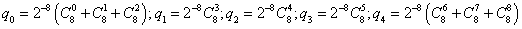ID 772987
Date 12/04/2020
Public

## Count-the-1's Test (Stream of Specific Bytes)

### Test Purpose

The test evaluates the randomness of the overlapping random five-letter words sequence. The five-letter words have the specified distribution of the probabilities of obtaining the specified letter. The test forms the random letters from the integer output of a BRNG. The test selects only 8 sequential bits from each element, starting with a certain fixed bit s.

### First Level Test

The test selects the ds, ds+1, ..., ds+7 bits determining the next random byte from each element of the integer output, where 0 £ s £ NB - 8 (see the table below). The number of 1’s in every random byte should have a binominal distribution with m = 8, p = 1/2 parameters. Therefore, the probability of getting k 1’s in a byte is equal to 2-8C8k. The first level test regards a random number that takes five possible values:

c = 0, if the number of 1’s in a random byte is less than three,

c = 1, if the number of 1’s in a random byte is three,

c = 2, if the number of 1’s in a random byte is four,

c = 3, if the number of 1’s in a random byte is five,

c = 4, if the number of 1’s in a random byte is more than five.

The probability distribution of c is the following:.

The test interprets c as a selection of a random letter from the alphabet {a, b, c, d, e} with the respective probabilities q0, q1, q2, q3, q4. Thus, the sequence of random bytes b0, b1, b2, ... corresponds with the defined sequence of random letters l0, l1, l2, ... . The test forms overlapping words of length four: v1 = l1l2l3l4, v2 = l2l3l4l5, ... and length five: w1 = l1l2l3l4l5, w2 = l2l3l4l5l6, ... from this sequence. The test computes the frequencies of getting each of 625 of possible four-letter words and of 3,125 of possible five-letter words for 256,000 of the obtained words. According to these frequencies, the test makes the chi-square statistics V1 and V2 for the four- and five-letter words respectively. The test takes into account the covariance of the frequencies of the fallouts of four-letter and five-letter words and performs the chi-square test for the V2 -V1 statistic. The V2 -V1 statistic is asymptotically normal with a mean a = 2500 and standard distribution s = 70.71. The result of the first level test is the p-value.

In the table below, NB stands for the number of bits required to represent a random number in integer arithmetic, WS stands for the machine word size, in bits, used in integer random number generation.

BRNG Integer Output Interpretation
MCG31m1

Array of 32-bit integers. Each 32-bit integer uses the following bits:

0-30. NB=31, WS=32.

R250

Array of 32-bit integers. Each 32-bit integer uses the following bits:

0-31. NB=32, WS=32.

MRG32k3a

Array of 32-bit integers. Each 32-bit integer uses the following bits:

0-31. NB=32, WS=32.

MCG59

Array of 64-bit integers. Each 64-bit integer uses the following bits:

0-58. NB=59, WS=64.

WH

Array of quadruples of 32-bit integers. Each 32-bit integer uses the following bits:

0-23. NB=24, WS=32.

MT19937

Array of 32-bit integers. Each 32-bit integer uses the following bits:

0-31. NB=32, WS=32.

MT2203

Array of 32-bit integers. Each 32-bit integer uses the following bits:

0-31. NB=32, WS=32.

SFMT19937

Array of quadruples of 32-bit integers. Each 32-bit integer uses the following bits:

0-31. NB=32, WS=32.

PHILOX4X32X10

Array of 32-bit integers. Each 32-bit integer uses the following bits:

0-31. NB=32, WS=32.

ARS5

Array of 32-bit integers. Each 32-bit integer uses the following bits:

0-31. NB=32, WS=32.

### Second Level Test

The second level test performs the first level test ten times for the fixed 0 £ s £ NB - 8. The test applies the Kolmogorov-Smirnov goodness-of-fit test with Anderson-Darling statistics to the obtained p-values of pj, j = 1, 2, ..., 10. If the resulting p-value is p < 0.05 or p > 0.95, the test fails for s.

### Final Result Interpretation

The second level test performs ten times for each of 0 £ s £ NB - 8. The test computes the FAIL percentage of the failed second level tests. The final result is the minimal for 0 £ s £ NB - 8 percentage of the failed tests FAIL = min(FAIL0, FAIL1, ..., FAILNB-8). The acceptable result is the value of FAIL < 50%. Therefore, the test determines whether it is possible to select at least 8 random bits from each element of the integer output of the generator.

### Tested Generators

Function Name Application
vsRngUniform
not applicable
vdRngUniform
not applicable
viRngUniform
not applicable
viRngUniformBits
applicable

The test checks each of the four elements separately for the WH and SFMT19937 generators.